# 机器学习系列(1)_逻辑回归初步

## 2、由来

要说逻辑回归，我们得追溯到线性回归，想必大家对线性回归都有一定的了解，即对于多维空间中存在的样本点，我们用特征的线性组合去拟合空间中点的分布和轨迹。如下图所示：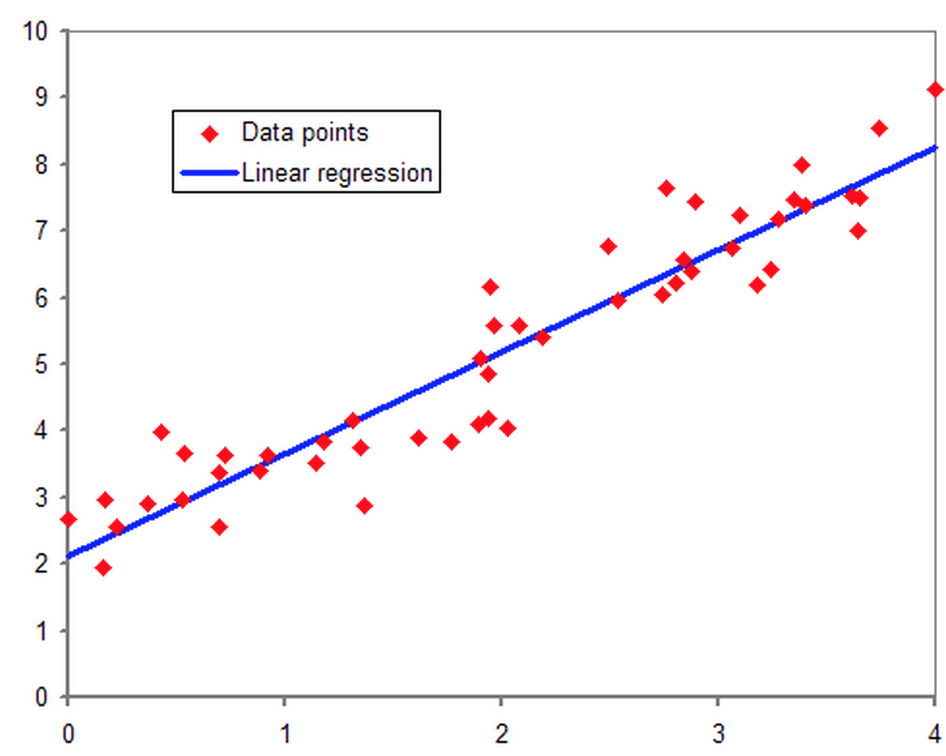线性回归能对连续值结果进行预测，而现实生活中常见的另外一类问题是，分类问题。最简单的情况是是与否的二分类问题。比如说医生需要判断病人是否生病，银行要判断一个人的信用程度是否达到可以给他发信用卡的程度，邮件收件箱要自动对邮件分类为正常邮件和垃圾邮件等等。

当然，我们最直接的想法是，既然能够用线性回归预测出连续值结果，那根据结果设定一个阈值是不是就可以解决这个问题了呢？事实是，对于很标准的情况，确实可以的，这里我们套用Andrew Ng老师的课件中的例子，下图中X为数据点肿瘤的大小，Y为观测结果是否是恶性肿瘤。通过构建线性回归模型，如hθ(x)所示，构建线性回归模型后，我们设定一个阈值0.5，预测hθ(x)≥0.5的这些点为恶性肿瘤，而hθ(x)<0.5为良性肿瘤。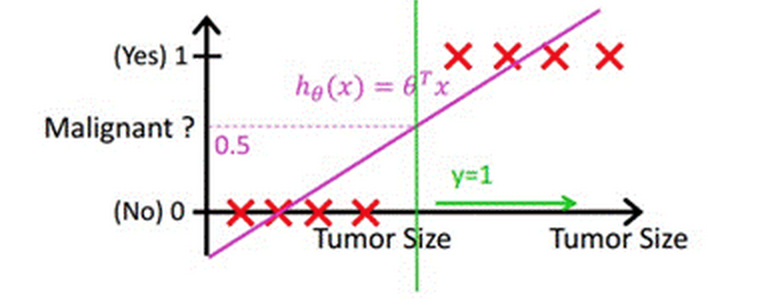但很多实际的情况下，我们需要学习的分类数据并没有这么精准，比如说上述例子中突然有一个不按套路出牌的数据点出现，如下图所示：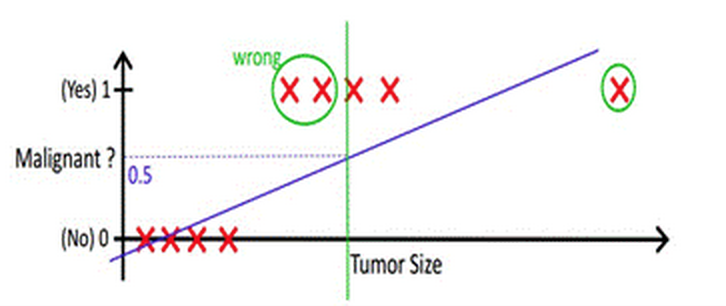你看，现在你再设定0.5，这个判定阈值就失效了，而现实生活的分类问题的数据，会比例子中这个更为复杂，而这个时候我们借助于线性回归+阈值的方式，已经很难完成一个鲁棒性很好的分类器了。

在这样的场景下，逻辑回归就诞生了。它的核心思想是，如果线性回归的结果输出是一个连续值，而值的范围是无法限定的，那我们有没有办法把这个结果值映射为可以帮助我们判断的结果呢。而如果输出结果是 (0,1) 的一个概率值，这个问题就很清楚了。我们在数学上找了一圈，还真就找着这样一个简单的函数了，就是很神奇的sigmoid函数(如下)：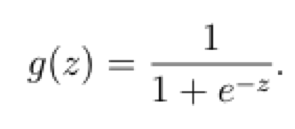如果把sigmoid函数图像画出来，是如下的样子：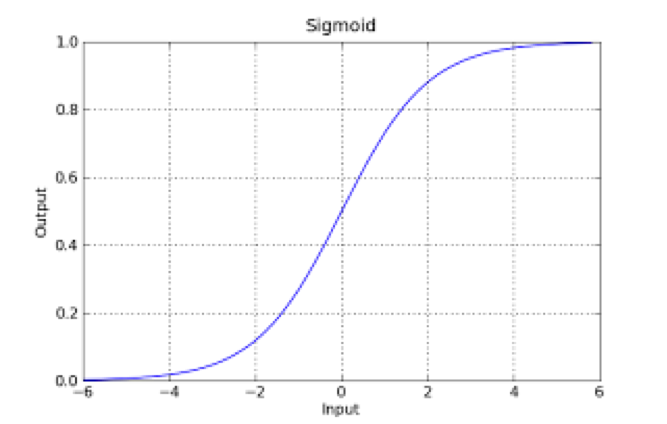Sigmoid Logistic Function

从函数图上可以看出，函数y=g(z)在z=0的时候取值为1/2，而随着z逐渐变小，函数值趋于0，z逐渐变大的同时函数值逐渐趋于1，而这正是一个概率的范围。

所以我们定义线性回归的预测函数为Y=WTX，那么逻辑回归的输出Y= g(WTX)，其中y=g(z)函数正是上述sigmoid函数(或者简单叫做S形函数)。

## 3、判定边界

我们现在再来看看，为什么逻辑回归能够解决分类问题。这里引入一个概念，叫做判定边界，可以理解为是用以对不同类别的数据分割的边界，边界的两旁应该是不同类别的数据。

从二维直角坐标系中，举几个例子，大概是如下这个样子：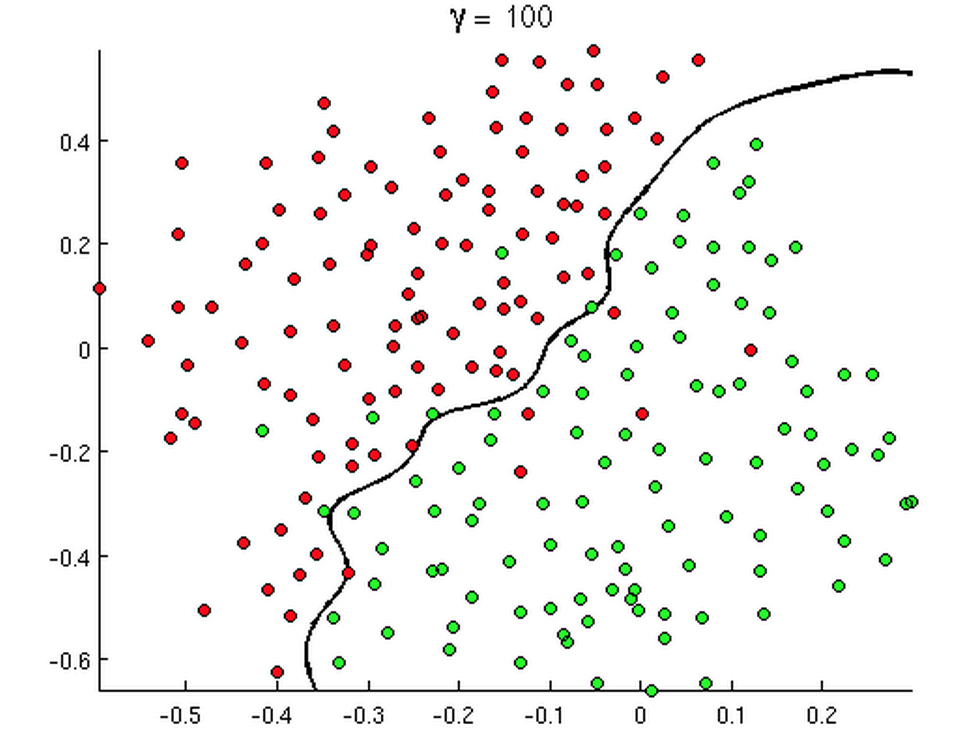有时候是这个样子：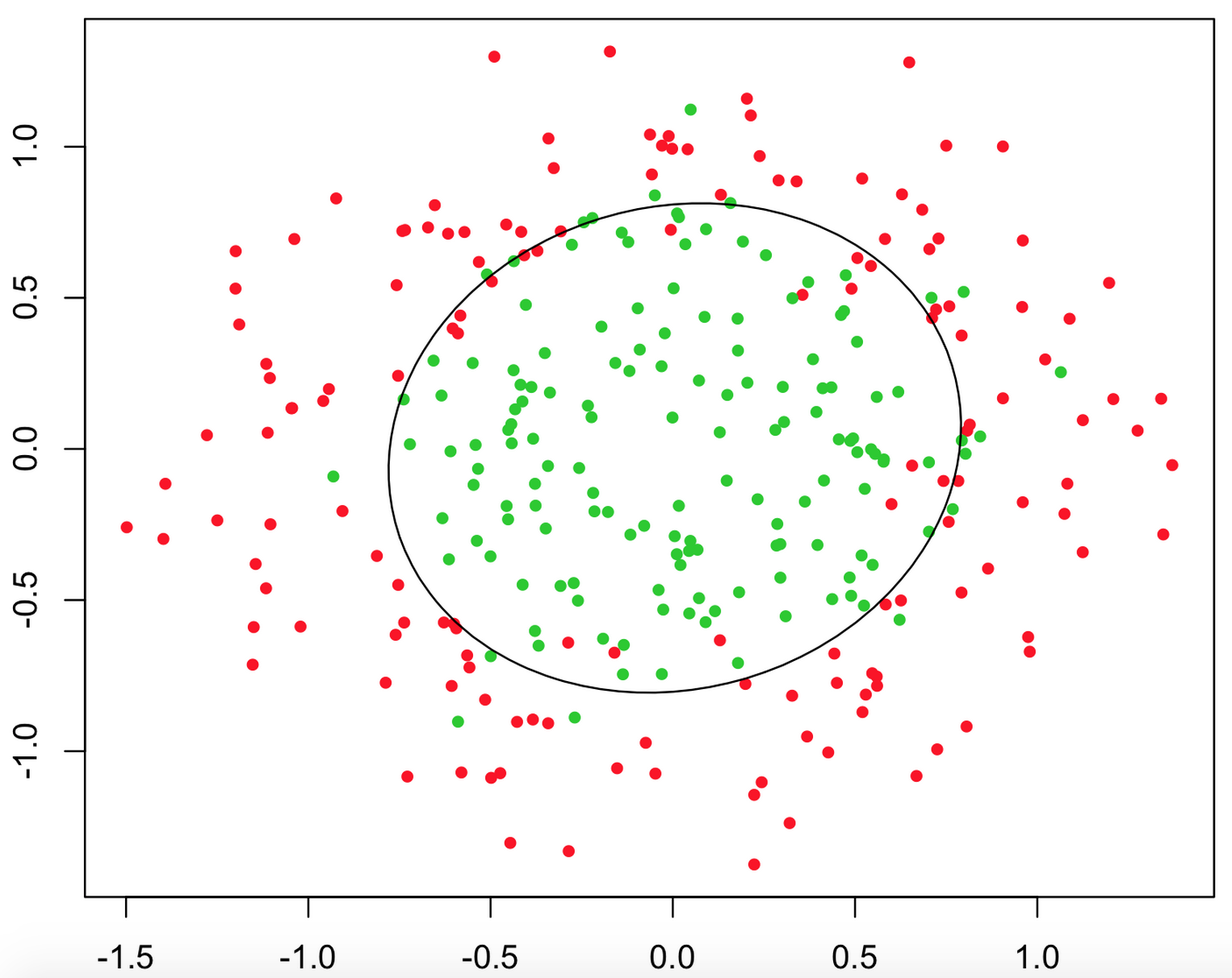甚至可能是这个样子：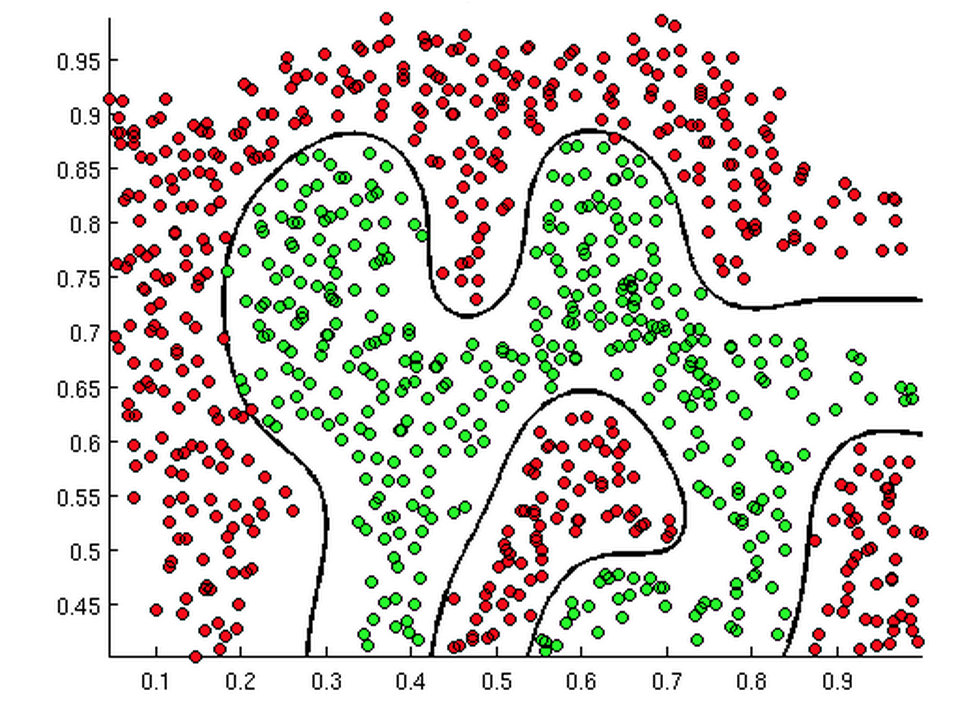上述三幅图中的红绿样本点为不同类别的样本，而我们划出的线，不管是直线、圆或者是曲线，都能比较好地将图中的两类样本分割开来。这就是我们的判定边界，下面我们来看看，逻辑回归是如何根据样本点获得这些判定边界的。

我们依旧借用Andrew Ng教授的课程中部分例子来讲述这个问题。

回到sigmoid函数，我们发现：

当 g(z)0.5 时,  z0 ;

对于 hθ (x)=g(θTX)0.5, 则 θTX0, 此时意味着预估y=1;

反之，当预测y = 0时， θTX<0;

所以我们认为θTX =0是一个决策边界，当它大于0或小于0时，逻辑回归模型分别预测不同的分类结果。

先看第一个例子hθ(x)=g(θ01X12X2)，其中 θ0 ,θ1 ,θ2分别取-3, 1, 1。则当−3+X1+X20时, y = 1; 则X1+X2=3是一个决策边界，图形表示如下，刚好把图上的两类点区分开来：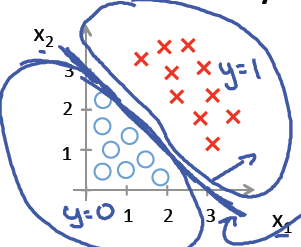例1只是一个线性的决策边界，当 hθ (x)更复杂的时候，我们可以得到非线性的决策边界，例如：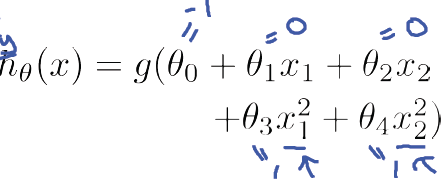这时当x12+x22≥1时，我们判定y=1，这时的决策边界是一个圆形，如下图所示：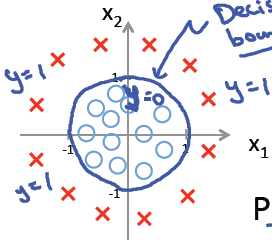所以我们发现，理论上说，只要我们的 hθ (x)设计足够合理，准确的说是g(θTx)中θTx足够复杂，我们能在不同的情形下，拟合出不同的判定边界，从而把不同的样本点分隔开来。

## 4、代价函数与梯度下降

我们通过对判定边界的说明，知道会有合适的参数θ使得θTx=0成为很好的分类判定边界，那么问题就来了，我们如何判定我们的参数θ是否合适，有多合适呢？更进一步，我们有没有办法去求得这样的合适参数θ呢？

这就是我们要提到的代价函数与梯度下降了。

所谓的代价函数Cost Function，其实是一种衡量我们在这组参数下预估的结果和实际结果差距的函数，比如说线性回归的代价函数定义为: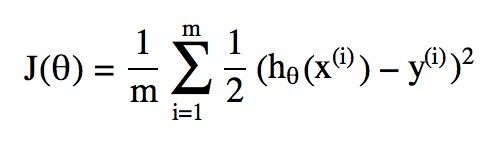因此，上述的Cost Function对于逻辑回归是不可行的，我们需要其他形式的Cost Function来保证逻辑回归的成本函数是凸函数。

我们跳过大量的数学推导，直接出结论了，我们找到了一个适合逻辑回归的代价函数: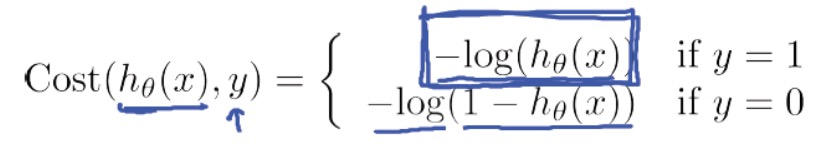Andrew Ng老师解释了一下这个代价函数的合理性，我们首先看当y=1的情况：如果我们的类别y = 1, 而判定的 hθ (x)=1，则Cost = 0，此时预测的值和真实的值完全相等，代价本该为0；而如果判断hθ(x)0，代价->∞，这很好地惩罚了最后的结果。

而对于y=0的情况，如下图所示，也同样合理：下面我们说说梯度下降，梯度下降算法是调整参数θ使得代价函数J(θ)取得最小值的最基本方法之一。从直观上理解，就是我们在碗状结构的凸函数上取一个初始值，然后挪动这个值一步步靠近最低点的过程，如下图所示：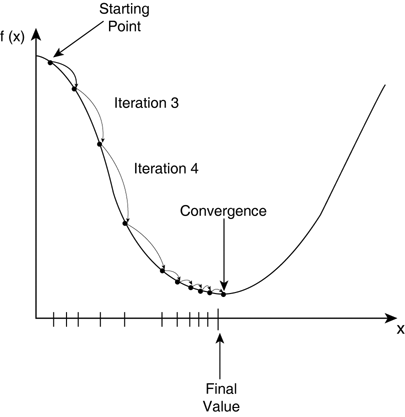我们先简化一下逻辑回归的代价函数：从数学上理解，我们为了找到最小值点，就应该朝着下降速度最快的方向(导函数/偏导方向)迈进，每次迈进一小步，再看看此时的下降最快方向是哪，再朝着这个方向迈进，直至最低点。

用迭代公式表示出来的最小化 J(θ ) 的梯度下降算法如下：## 5、代码与实现

我们来一起看两个具体数据上做逻辑回归分类的例子，其中一份数据为线性判定边界，另一份为非线性。

示例1。

第一份数据为data1.txt，部分内容如下：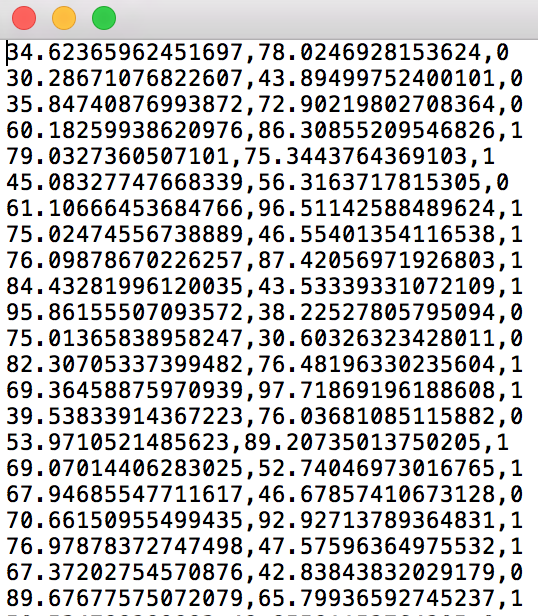我们先来看看数据在空间的分布，代码如下。

from numpy import loadtxt, where
from pylab import scatter, show, legend, xlabel, ylabel

X = data[:, 0:2]
y = data[:, 2]

pos = where(y == 1)
neg = where(y == 0)
scatter(X[pos, 0], X[pos, 1], marker='o', c='b')
scatter(X[neg, 0], X[neg, 1], marker='x', c='r')
xlabel('Feature1/Exam 1 score')
ylabel('Feature2/Exam 2 score')
legend(['Fail', 'Pass'])
show()


得到的结果如下：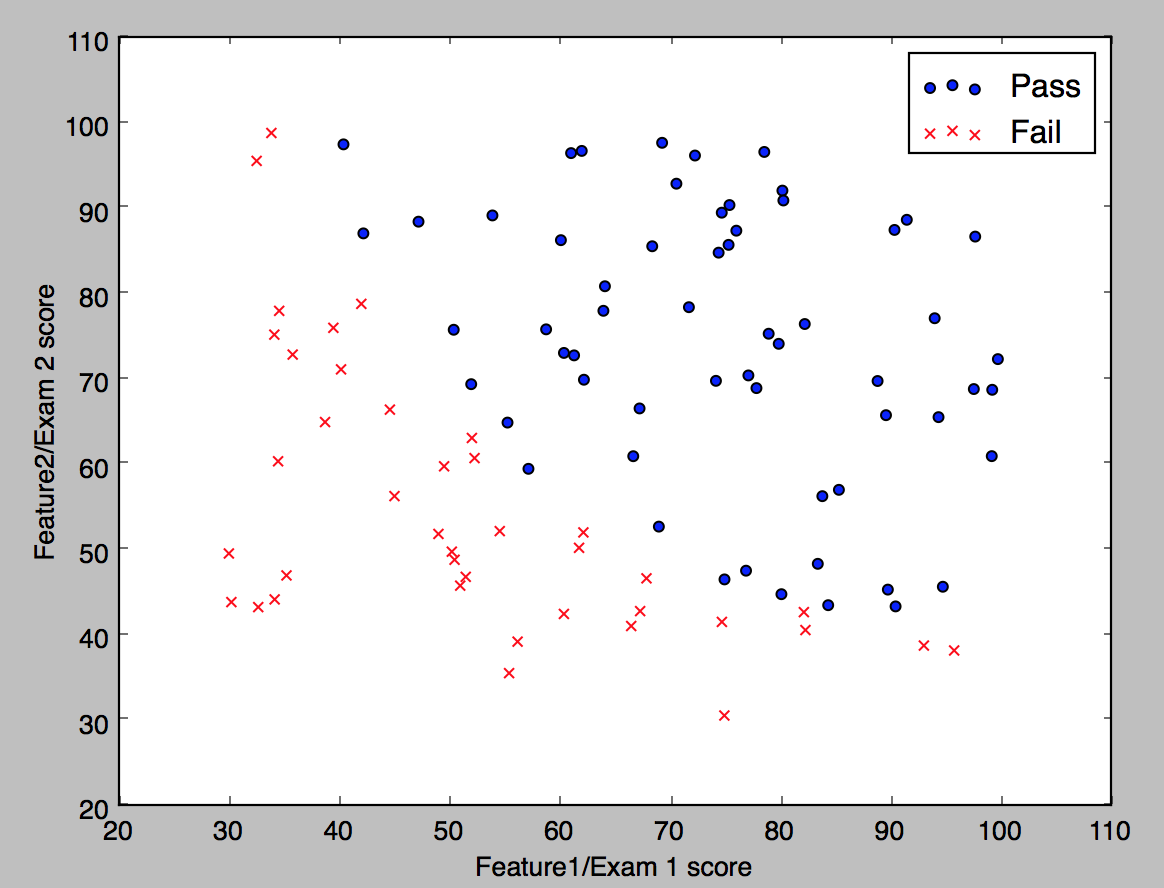下面我们写好计算sigmoid函数、代价函数、和梯度下降的程序：

def sigmoid(X):
'''Compute sigmoid function '''
den =1.0+ e **(-1.0* X)
gz =1.0/ den
return gz
def compute_cost(theta,X,y):
'''computes cost given predicted and actual values'''
m = X.shape#number of training examples
theta = reshape(theta,(len(theta),1))

J =(1./m)*(-transpose(y).dot(log(sigmoid(X.dot(theta))))- transpose(1-y).dot(log(1-sigmoid(X.dot(theta)))))

#optimize.fmin expects a single value, so cannot return grad
theta.shape =(1,3)
h = sigmoid(X.dot(theta.T))
delta = h - y
for i in range(l):
sumdelta = delta.T.dot(X[:, i])
theta.shape =(3,)


我们用梯度下降算法得到的结果判定边界是如下的样子：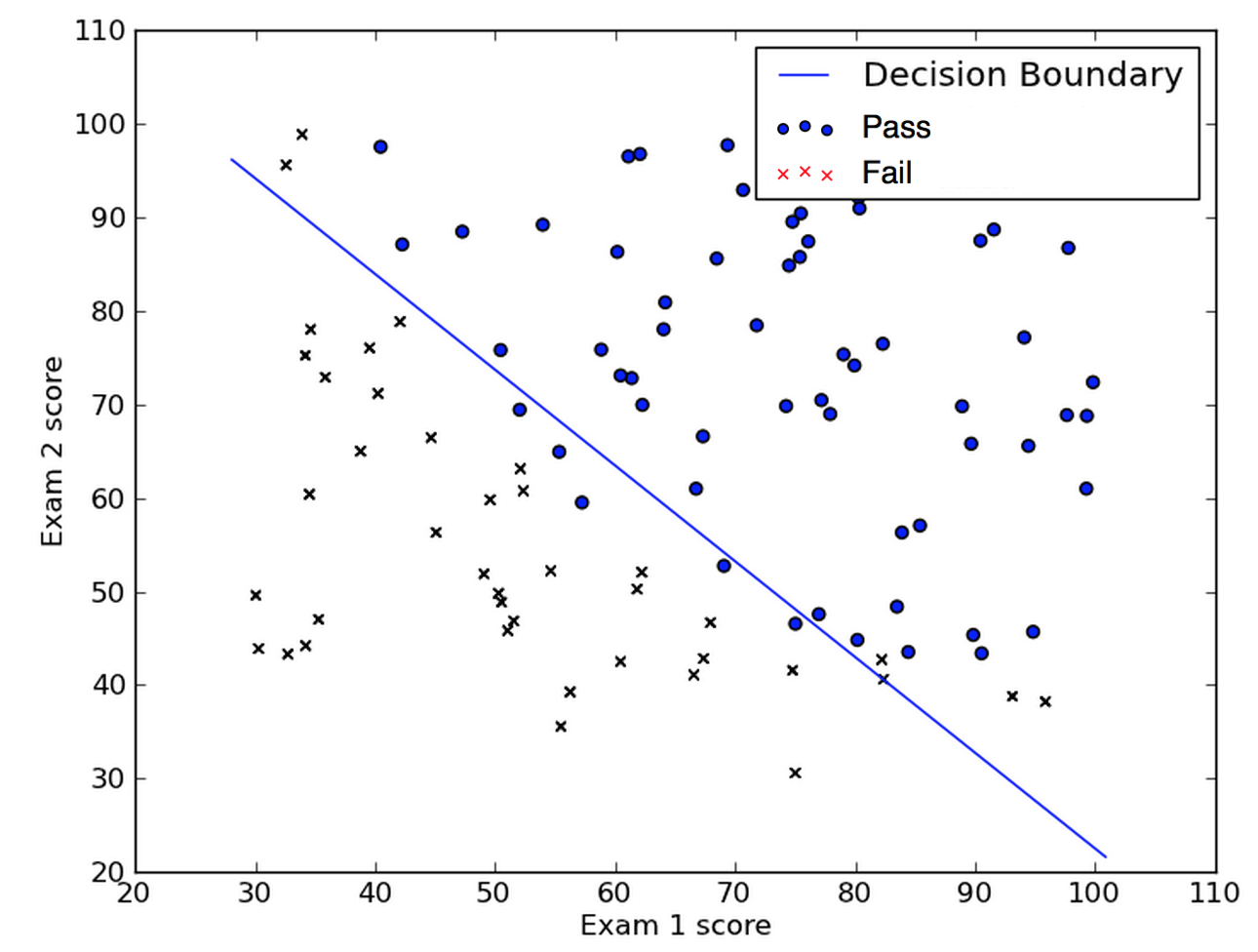最后我们使用我们的判定边界对training data做一个预测，然后比对一下准确率：

def predict(theta, X):
'''Predict label using learned logistic regression parameters'''
m, n = X.shape
p = zeros(shape=(m,1))
h = sigmoid(X.dot(theta.T))
for it in range(0, h.shape):
if h[it]>0.5:
p[it,0]=1
else:
p[it,0]=0
return p
#Compute accuracy on our training set
p = predict(array(theta), it)
print'Train Accuracy: %f'%((y[where(p == y)].size / float(y.size))*100.0)

计算出来的结果是89.2%

示例2.

第二份数据为data2.txt，部分内容如下：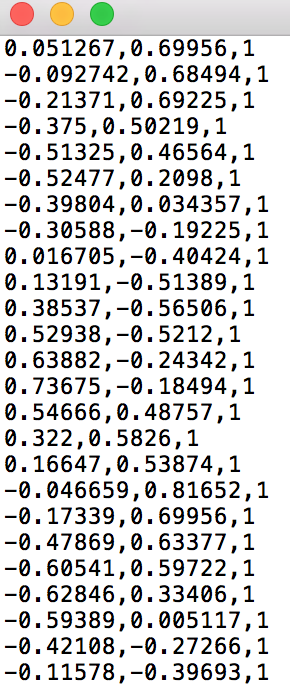我们同样把数据的分布画出来，如下：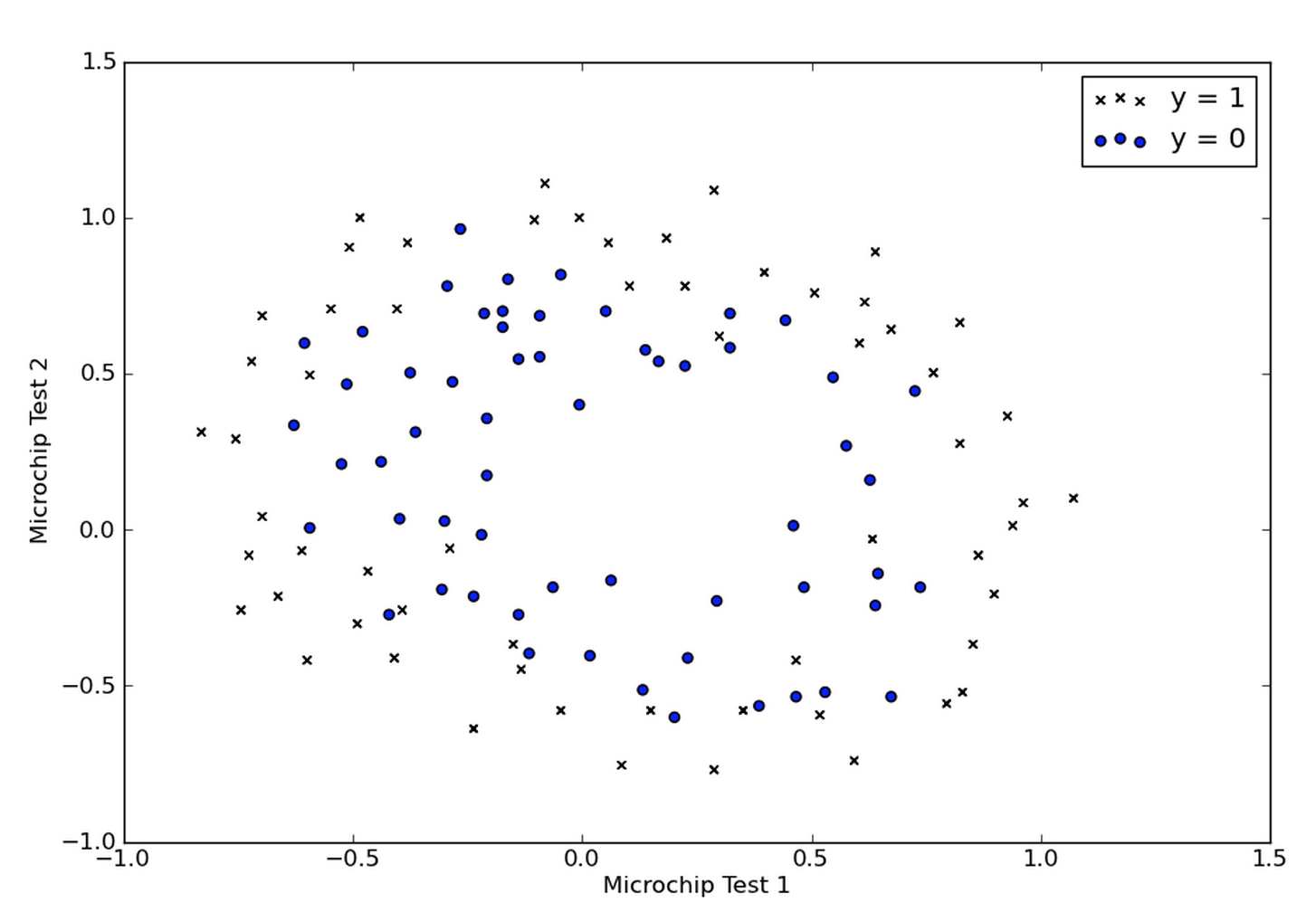我们发现在这个例子中，我们没有办法再用一条直线把两类样本点近似分开了，所以我们打算试试多项式的判定边界，那么我们先要对给定的两个feature做一个多项式特征的映射。比如说，我们做了如下的一个映射：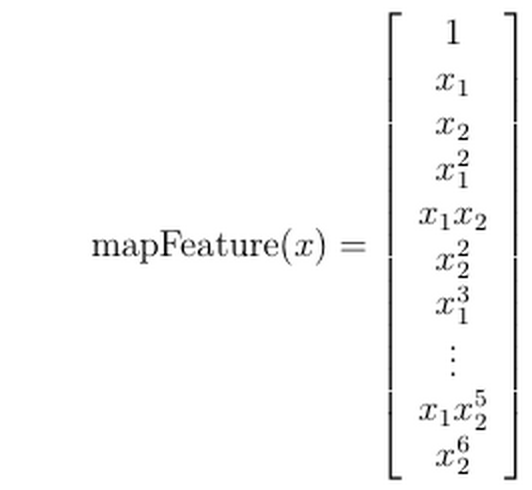代码如下：

def map_feature(x1, x2):
'''
Maps the two input features to polonomial features.
Returns a new feature array with more features of
X1, X2, X1 ** 2, X2 ** 2, X1*X2, X1*X2 ** 2, etc...
'''
x1.shape =(x1.size,1)
x2.shape =(x2.size,1)
degree =6
mapped_fea = ones(shape=(x1[:,0].size,1))
m, n = mapped_fea.shape
for i in range(1, degree +1):
for j in range(i +1):
r =(x1 **(i - j))*(x2 ** j)
mapped_fea = append(mapped_fea, r, axis=1)
return mapped_fea
mapped_fea = map_feature(X[:,0], X[:,1])

接着做梯度下降：

def cost_function_reg(theta, X, y, l):
'''Compute the cost and partial derivatives as grads
'''
h = sigmoid(X.dot(theta))
thetaR = theta[1:,0]
J =(1.0/ m)*((-y.T.dot(log(h)))-((1- y.T).dot(log(1.0- h)))) \
+(l /(2.0* m))*(thetaR.T.dot(thetaR))
delta = h - y
sum_delta = delta.T.dot(X[:,1])
XR = X[:,1:X.shape]
sum_delta = delta.T.dot(XR)
grad =(1.0/ m)*(sum_delta + l * thetaR)
return J.flatten(), out.T.flatten()
m, n = X.shape
y.shape =(m,1)
it = map_feature(X[:,0], X[:,1])
#Initialize theta parameters
initial_theta = zeros(shape=(it.shape,1))
#Use regularization and set parameter lambda to 1
l =1
# Compute and display initial cost and gradient for regularized logistic
# regression
cost, grad = cost_function_reg(initial_theta, it, y, l)
def decorated_cost(theta):
return cost_function_reg(theta, it, y, l)
print fmin_bfgs(decorated_cost, initial_theta, maxfun=500)

接着在数据点上画出判定边界：

#Plot Boundary
u = linspace(-1,1.5,50)
v = linspace(-1,1.5,50)
z = zeros(shape=(len(u), len(v)))
for i in range(len(u)):
for j in range(len(v)):
z[i, j]=(map_feature(array(u[i]), array(v[j])).dot(array(theta)))
z = z.T
contour(u, v, z)
title('lambda = %f'% l)
xlabel('Microchip Test 1')
ylabel('Microchip Test 2')
legend(['y = 1','y = 0','Decision boundary'])
show()
def predict(theta, X):
'''Predict whether the label
is 0 or 1 using learned logistic
regression parameters '''
m, n = X.shape
p = zeros(shape=(m,1))
h = sigmoid(X.dot(theta.T))
for it in range(0, h.shape):
if h[it]>0.5:
p[it,0]=1
else:
p[it,0]=0
return p
#% Compute accuracy on our training set
p = predict(array(theta), it)
print'Train Accuracy: %f'%((y[where(p == y)].size / float(y.size))*100.0)

得到的结果如下图所示：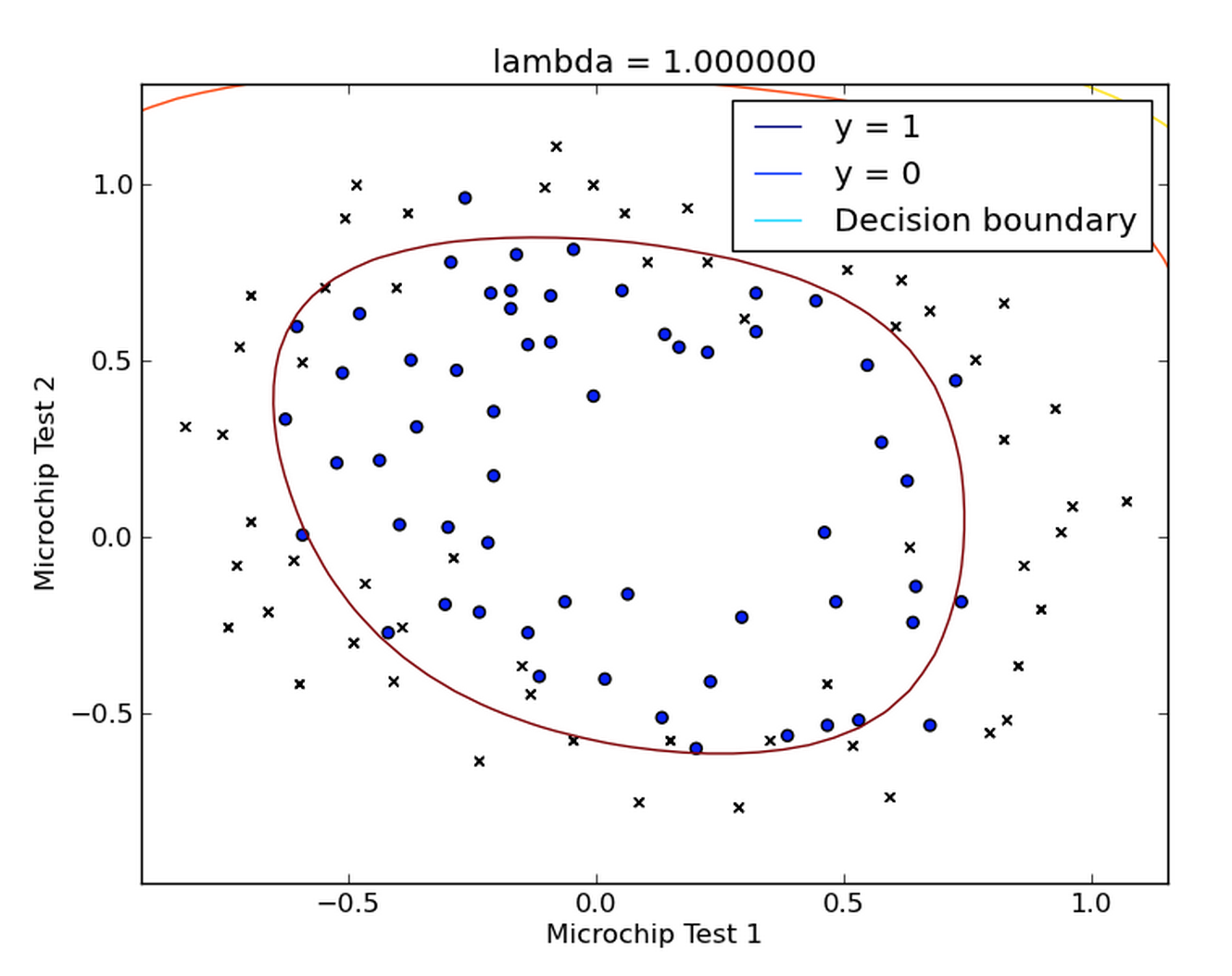我们发现我们得到的这条曲线确实将两类点区分开来了。

## 6、总结

最后我们总结一下逻辑回归。它始于输出结果为有实际意义的连续值的线性回归，但是线性回归对于分类的问题没有办法准确而又具备鲁棒性地分割，因此我们设计出了逻辑回归这样一个算法，它的输出结果表征了某个样本属于某类别的概率。

逻辑回归的成功之处在于，将原本输出结果范围可以非常大的θTX 通过sigmoid函数映射到(0,1)，从而完成概率的估测。

而直观地在二维空间理解逻辑回归，是sigmoid函数的特性，使得判定的阈值能够映射为平面的一条判定边界，当然随着特征的复杂化，判定边界可能是多种多样的样貌，但是它能够较好地把两类样本点分隔开，解决分类问题。

求解逻辑回归参数的传统方法是梯度下降，构造为凸函数的代价函数后，每次沿着偏导方向(下降速度最快方向)迈进一小部分，直至N次迭代后到达最低点。

## 7、补充

本文的2份数据可在http://pan.baidu.com/s/1pKxJl1p上下载到，分别为data1.txt和data2.txt，欢迎大家自己动手尝试。

关于逻辑回归的完整ipython notebook示例代码可以在我的github上(https://github.com/HanXiaoyang/ML_examples/tree/master/logistic_regression)下载到，欢迎指正。

10-11
02-07
10-1194
11-301406
04-071513
11-05829
11-11870
03-08128
07-22894
04-252971
05-20424
08-02166
04-01157
05-033255
09-23920
03-271058
12-08137
07-24528
04-143543
12-0886
04-103108

### “相关推荐”对你有帮助么？

•非常没帮助
•没帮助
•一般
•有帮助
•非常有帮助被折叠的  条评论 为什么被折叠?到【灌水乐园】发言¥2 ¥4 ¥6 ¥10 ¥20余额支付 (余额：-- )扫码支付获取中扫码支付点击重新获取扫码支付1.余额是钱包充值的虚拟货币，按照1:1的比例进行支付金额的抵扣。
2.余额无法直接购买下载，可以购买VIP、C币套餐、付费专栏及课程。余额充值